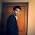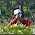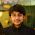# Oversampling for rare event

This tutorial describes the effects of oversampling on a rare event model. Suppose you are building a logistic regression model in which % of events (desired outcome) is very low (less than 1%). You need to make a treatment to make the model robust so that enough events would be used to train the model. Oversampling is one of the treatment to deal rare-event problem.Effects of Oversampling
Oversampling

Suppose you are working on a retail customer attrition (churn) problem for a telecom company. You started building a logistic regression model in which target (dependent) variable is defined as whether a customer is active or not. If a customer is NOT active, it is 1 in target variable. Otherwise it is 0. You calculated attrition percentage (i.e. mean of the target variable) and found it's 1% of 10,000 customer base. It means there are 9900 active customers and 100 attritors in 10k cases of your target variable. Since the distribution of target variable is highly skewed, you need to oversample the event (attritors). By oversampling, it means decreasing the volume of non-events so that proportion of events and non-events gets balanced or less skewed.
You take a small proportion from non-event cases and a large proportion (or all the records) from the relatively few event cases

When should we perform Oversampling

In logistic regression, it is required to have a minimum of 10 events per independent variable. Many people get confused about this thumb rule of having minimum number of independent variables (predictors). It's a commonly asked question ' Does this rule apply before variable selection or after variable selection?' The answer is : Suppose you have 30 events. You are running a stepwise selection method for variable selection (i.e. adding a variable one by one and checking the significance level of the previous variables at every addition). This rule would be applied on all the candidate variables in the stepwise algorithm but you should limit the algorithm to consider only 3 independent variables. Some researchers do not follow this rule strictly and do oversampling even if this rule is met. It is advisable to build two models (with or without oversampling) and test the model on non-oversampling population and compare the accuracy of the models.

Terminologies related to oversampling

Prior Probability : The prior probability is a probability of events before you have oversampled data.

Posterior Probability : The posterior probability is a probability of events after you have oversampled data. It is a conditional distribution because it conditions on the observed data.

Posterior probability is normally calculated by updating the prior probability by using Bayes' theorem.

How to perform Oversampling with SAS

Method I : With PROC SURVEYSELECT
proc sort data = full;
by y;
run;
proc surveyselect data = full out = sub method = srs n = (100,100) seed = 9876;
strata y;
run;
In the code above, we are performing stratified sampling. The option n = (number of 0s you want to keep, number of 1s you want to keep). Instead, you can use rate option - rate = (50,50). It means you want to retain 50% of 0s and 50% of 1s.

Method II : Without PROC SURVEYSELECT
data sub;
set full;
if y=1 or (y=0 and ranuni(75302)<1/9) then output;
run;
Note : 1/9 means 10% of events and 90% non-events in the original data (before sampling). After running the above code, distribution of events and non-events would be 50:50.

Effect of oversampling
1. Oversampling does not effect the slopes (parameter estimates), but it effects the intercepts (make it too high). In other words, parameter estimates remain same after sampling but intercepts increases very much after sampling.
2. Predicted probabilities are affected as it is calculated taking both paramter estimates and intercept (incorrect intercept as stated above). It increases after sampling as intercept is overestimated.
3. Oversampling does not affect sensitivity or specificity measures but false positive and negative rates are affected.
4. ROC curve is not affected by oversampling.
5. Oversampling does not affect rank ordering (sorting based on predicted probability) because adjusting oversampling is just a linear transformation. Hence, it does not affect Gain and Lift charts if you score on out of time sample or unsampled validation dataset. However, if you compare lift of unsampled and sampled data of training dataset, gain charts and lift charts are affected as proportion of events got changed. For example, predicted probability score is 80% in one observation. After oversampling, ratio is 50:50. The lift on the sampled data is 80%/50% = 1.6. After adjusting probability, the adjusted probability score is 30.8%. The lift on the original data is 3.08 (30.8% / 10%).

Correcting Confusion Matrix

Suppose, π0 is the proportion of non-events before sampling . π1 is the proportion of events before sampling. ρ1 is the proportion of events after sampling. ρ0 is the proportion of non-events after sampling.
True proportion of true positives = π1 * sensitivity.
True proportion of true negatives = π0 * specificity
True proportion of false positives = π0 * (1 - specificity)
True proportion of false negatives = π1 * (1 - sensitivity)
Note : When you correct for oversampling, you make the probabilities much, much smaller.

Correcting intercept and predicted probability

1. Correct Intercept - Offset Method

p1: the population rate (before oversampling) - Let's say 1%.
r1: the sample rate (post oversampling - 10%)
α1 is the intercept from oversampled data.

The intercept term for final model α when scoring non sampled population -
α= α1 + log ((p1(1-r1))/(1-p1) r1) where log represents the natural logarithm (loge).

2. Correct probability- Weight Method

In the equation below, P_1 denotes predicted probability for an event, P_0 denotes predicted probability for non-event.

Step 1 :  A =   P_1 / (Oversampled % of events / Original % of events)
Step 2 :  B  =  P_0 / (Oversampled % of non-events / Original % of non-events)
Step 3 :  Adj_P_1 = A / (A+B)
Step 4 :  Adj_P_0 = B / (A+B)

Solve the above equation :

Adj_P_1 = 1/(1+((1/original % of events)-1)/((1/oversampled %  of events)-1)*[(1/P_1)-1])
Adj_P_0 = 1/(1+((1/original % of non-events)-1)/((1/oversampled %  of non-events)-1)*[(1/P_0)-1])

Before Sampling - 1 - 5% and 0 -95%
Post Sampling -     1 - 50% and 0 -50%

Note : You do not need to adjust oversampling if your goal is to select the top 30% customers based on their high predicted probability. It is because it is just a linear transformation and it does not affect rank ordering. It should be performed only when you need to know the “correct” probability of customers.

I. Implementing Offset Method in SAS :

You can use the PRIOREVENT= option in the SCORE statement to specify the prior event probability.

proc logistic data=training;
model attrition(event='1')= Fees Balance Withdrawal Interest;
score data=valid out=scored priorevent=0.05;
run;

Note : 0.05 means the 5% of the target event before sampling.

II. Implementing Sampling weights in SAS :

Sampling weights adjust the data so that it better represents the true population.

Sampling weight for events = (proportion of events before sampling / proportion of events after sampling)

Sampling weight for non-events = (proportion of non-events before sampling / proportion of non-events after sampling)

You can use the WEIGHT statement in PROC LOGISTIC to weight each observation in the input data set by the value of the WEIGHT variable.

%let priorprob = 0.05;

proc sql noprint;
select mean(attrition) into :postprob from training;
quit;

data training1;
set training;
sampwt=((1-&priorprob )/(1-&postprob))*(attrition=0)+(&priorprob /&postprob)*(attrition=1);
run;

proc logistic data=training1;
weight sampwt;
model attrition(event='1')= Fees Balance Withdrawal Interest;
score data=valid out=scored;
run;

Note : priorprob is probability of an event before sampling. attrition is a dependent variable in this model.Related PostsDeepanshu founded ListenData with a simple objective - Make analytics easy to understand and follow. He has over 8 years of experience in data science. During his tenure, he has worked with global clients in various domains like Banking, Insurance, Telecom and Human Resource.

11 Responses to "Oversampling for rare event"
1.Deepanshu it helped a lot!!

2.Very well explained. Thanks

3.Hi, I have come across similar problem where I have 1.4 % churn rate (event) for around 3 million obs. I have taken 50-50 (all events and some non events). So in this case is it correct to use priorevent=0.016 in the score statement ( because my event rate was 1.6% before over sampling )?. Another question, if I do oversampling on training data and NOT on validation data, wouldn't event rate be very low in the validation dataset for sas to do validation? Many thanks.

1.Yes, priorevent = 0.016 is correct. The idea of using validation dataset is to validate the model and fitting equation derived from the training dataset on validation dataset. You have built your model on training data and now you are checking whether model works well on data outside training. If you do oversampling on validation data as well, it would NOT be a right method of validation of your model. It is because the real desired outcome rate (event rate) is 1.6% which you are trying to predict for the future population. Hope it helps!

2.Hi Deepanshu,

Does this mean that you oversample AFTER you split your train and validation data?

4.Thanks, this is useful.

Another question, does event rate matter if you have enough volume of events in the model? I am working on Churn model for telecom (as you have given the example), churn (event) rate is 0.7% but I have around 10,000 event volume for around 1 million observations. I am am testing around 20 variables in the model and final model has around 10 variables. My understanding is that if you have enough Event volume like in this case 10K, based on number of independent variables, low event rate should not matter?

1.yes, your understanding is correct. Low event rate does not matter if you have enough events dependending on the number of variables. This rule applies only to Logistic Regression. It's not safe to generalize for all the algorithms.

2.Cheers Deepanshu

5.Hi Deepanshu, If I have a case where I am using sample of 150k from the base and my churn rate is 1%, so 1500 cases of churners (events), do I really need to oversample if I am testing around 30 variables and final model has <20 variables. Also, as my probabilities are very low, my confusion matrix is super screwed at 0.4 cut off. How do I explain this ?

6.Nice work Deepanshu. I just had a small question. Could you please elaborate on why does the beta coefficients of the covariates not change after the oversampling?

7.Can't weight option of proc logistic be used to handle such cases?

Next → ← Prev
Love this Post? Spread the Word!
Share